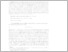Repository: Freie Universität Berlin, Math Department

# Fractal homogenization of multiscale interface problems

Heida, M. and Kornhuber, R. and Podlesny, J. (2020) Fractal homogenization of multiscale interface problems. Multiscale Modeling & Simulation, 18 (1). pp. 294-314. ISSN 1540-3459Preview

1MB

Official URL: https://doi.org/10.1137/18M1204759

## Abstract

Inspired by continuum mechanical contact problems with geological fault networks, we consider elliptic second order differential equations with jump conditions on a sequence of multiscale networks of interfaces with a finite number of nonseparating scales. Our aim is to derive and analyze a description of the asymptotic limit of infinitely many scales in order to quantify the effect of resolving the network only up to some finite number of interfaces, and to consider all further effects as homogeneous. As classical homogenization techniques are not suited for this kind of geometrical setting, we suggest a new concept, called fractal homogenization, to derive and analyze an asymptotic limit problem from a corresponding sequence of finite-scale interface problems. We provide an intuitive characterization of the corresponding fractal solution space in terms of generalized jumps and gradients together with continuous embeddings into \$L^2\$ and \$H^s\$, \$s<1/2\$. We show existence and uniqueness of the solution of the asymptotic limit problem and exponential convergence of the approximating finite-scale solutions. Computational experiments involving a related numerical homogenization technique illustrate our theoretical findings.

Item Type: Article Mathematical and Computer Sciences > Mathematics > Applied MathematicsMathematical and Computer Sciences > Mathematics > Numerical Analysis Department of Mathematics and Computer Science > Institute of Mathematics 2144 Ekaterina Engel 05 Dec 2017 14:07 22 Feb 2022 17:35

Repository Staff Only: item control page